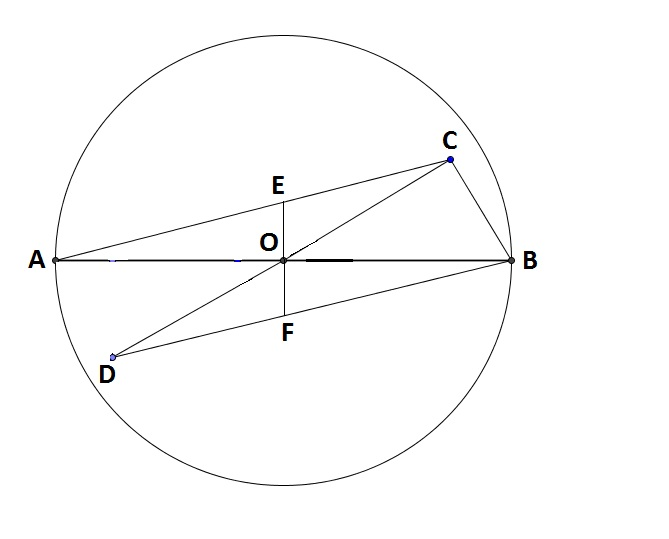# BdMO(Regional) 2012 Problem

Geometry Level 3In a circle, $AB=4$ is the diameter and $O$ is the centre. $C$ and $D$ are chosen such that $C,O,D$ are co-linear, $\angle COB= \angle AOD=30^\circ$, $OC=OD$ and $\angle BCO = 90^\circ$. The perpendicular on $AB$ through $O$ meets $AC$ at $E$ and $BD$ at $F$.

If $EF=\frac{a\sqrt{b}}{c}$ where $a,b,c$ are integers and $b,c$ are primes, find the value of $a+b+c$.

Note: $C$ and $D$ are not necessarily on the circumference of the circle.

×

Problem Loading...

Note Loading...

Set Loading...